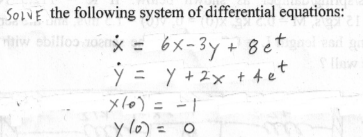Home / Expert Answers / Mechanical Engineering / solve-the-following-system-of-differential-equations-x-6x3y-8ety-y-2x-4etx-0-1y-0-pa187

# (Solved): SOLVE the following system of differential equations: x=6x3y+8ety=y+2x+4etx(0)=1y(0)= ...SOLVE the following system of differential equations:

We have an Answer from Expert

(A): Given the differential equations:

x' = 6x - 3y + 8et
y' = y + 2x + 4et
The given conditions are:

x(0) = -1
y(0) = 0

To solve the system, we can use the method of integrating factors.
Then, the integrating factor for the first equation is given by:

note that the differentiation had done to the above equation.

Multiply both sides of the first equation by   :

that means we have to substitute the equation in the first (x') differential equation.

e6tx' = 6e6tx - 3e6ty + 8et*e6t

e6tx' = 6e6tx - 3e6ty + 8e7t

We have an Answer from Expert